# NCERT Exemplar solutions for Class 11 Physics chapter 15 - Waves [Latest edition]

#### Chapters## Solutions for Chapter 15: Waves

Below listed, you can find solutions for Chapter 15 of CBSE NCERT Exemplar for Class 11 Physics.

Exercises
Exercises [Pages 105 - 112]

### NCERT Exemplar solutions for Class 11 Physics Chapter 15 Waves Exercises [Pages 105 - 112]

#### MCQ I

Exercises | Q 15.1 | Page 105

Water waves produced by a motor boat sailing in water are ______.

• neither longitudinal nor transverse.

• both longitudinal and transverse.

• only longitudinal.

• only transverse.

Exercises | Q 15.2 | Page 105

Sound waves of wavelength λ travelling in a medium with a speed of v m/s enter into another medium where its speed is 2v m/s. Wavelength of sound waves in the second medium is ______.

• λ

• λ/2

Exercises | Q 15.3 | Page 106

Speed of sound wave in air ______.

• is independent of temperature.

• increases with pressure.

• increases with increase in humidity.

• decreases with increase in humidity.

Exercises | Q 15.4 | Page 106

Change in temperature of the medium changes ______.

• frequency of sound waves.

• amplitude of sound waves.

• wavelength of sound waves.

• loudness of sound waves.

Exercises | Q 15.5 | Page 106

With propagation of longitudinal waves through a medium, the quantity transmitted is ______.

• matter.

• energy.

• energy and matter.

• energy, matter and momentum.

Exercises | Q 15.6 | Page 106

Which of the following statements are true for wave motion?

• Mechanical transverse waves can propagate through all mediums.

• Longitudinal waves can propagate through solids only.

• Mechanical transverse waves can propagate through solids only.

• Longitudinal waves can propagate through vacuum.

Exercises | Q 15.7 | Page 106

A sound wave is passing through air column in the form of compression and rarefaction. In consecutive compressions and rarefactions ______.

• density remains constant.

• Boyle’s law is obeyed.

• bulk modulus of air oscillates.

• there is no transfer of heat.

Exercises | Q 15.8 | Page 106

Equation of a plane progressive wave is given by y = 0.6 sin 2π (t - x/2). On reflection from a denser medium its amplitude becomes 2/3 of the amplitude of the incident wave. The equation of the reflected wave is ______.

• y = 0.6 sin 2π (t + x/2)

• y = - 0.4 sin 2π (t + x/2)

• y = 0.4 sin 2π (t + x/2)

• y = - 0.4 sin 2π (t - x/2)

Exercises | Q 15.9 | Page 107

A string of mass 2.5 kg is under a tension of 200 N. The length of the stretched string is 20.0 m. If the transverse jerk is struck at one end of the string, the disturbance will reach the other end in ______.

• one second

• 0.5 second

• 2 seconds

• data given is insufficient

Exercises | Q 15.10 | Page 107

A train whistling at constant frequency is moving towards a station at a constant speed V. The train goes past a stationary observer on the station. The frequency n ′ of the sound as heard by the observer is plotted as a function of time t (figure). Identify the expected curve.

•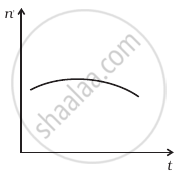•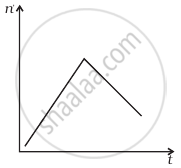•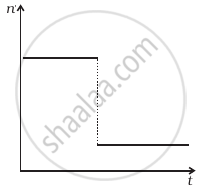•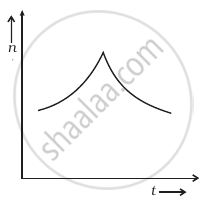#### MCQ II

Exercises | Q 15.11 | Page 108

A transverse harmonic wave on a string is described by y(x, t) = 3.0 sin (36t + 0.018x + π/4) where x and y are in cm and t is in s. The positive direction of x is from left to right.

1. The wave is travelling from right to left.
2. The speed of the wave is 20 m/s.
3. Frequency of the wave is 5.7 Hz.
4. The least distance between two successive crests in the wave is 2.5 cm.
Exercises | Q 15.12 | Page 108

The displacement of a string is given by y (x, t) = 0.06 sin (2πx/3) cos (120 πt) where x and y are in m and t in s. The length of the string is 1.5 m and its mass is 3.0 × 10−2 kg.

1. It represents a progressive wave of frequency 60 Hz.
2. It represents a stationary wave of frequency 60 Hz.
3. It is the result of superposition of two waves of wavelength 3 m, frequency 60 Hz each travelling with a speed of 180 m/s in opposite direction.
4. Amplitude of this wave is constant.
Exercises | Q 15.13 | Page 108

Speed of sound waves in a fluid depends upon ______.

1. directty on density of the medium.
2. square of Bulk modulus of the medium.
3. inversly on the square root of density.
4. directly on the square root of bulk modulus of the medium.
Exercises | Q 15.14 | Page 108

During propagation of a plane progressive mechanical wave ______.

1. all the particles are vibrating in the same phase.
2. amplitude of all the particles is equal.
3. particles of the medium executes S.H.M.
4. wave velocity depends upon the nature of the medium.
Exercises | Q 15.15 | Page 108

The transverse displacement of a string (clamped at its both ends) is given by y(x, t) = 0.06 sin (2πx/3) cos (120 πt). All the points on the string between two consecutive nodes vibrate with ______.

1. same frequency
2. same phase
3. same energy
4. different amplitude.
Exercises | Q 15.16 | Page 109

A train, standing in a station yard, blows a whistle of frequency 400 Hz in still air. The wind starts blowing in the direction from the yard to the station with a speed of 10 m/s. Given that the speed of sound in still air is 340 m/s ______.

1. the frequency of sound as heard by an observer standing on the platform is 400 Hz.
2. the speed of sound for the observer standing on the platform is 350 m/s.
3. the frequency of sound as heard by the observer standing on the platform will increase.
4. the frequency of sound as heard by the observer standing on the platform will decrease.
Exercises | Q 15.17 | Page 109

Which of the following statements are true for a stationary wave?

1. Every particle has a fixed amplitude which is different from the amplitude of its nearest particle.
2. All the particles cross their mean position at the same time.
3. All the particles are oscillating with same amplitude.
4. There is no net transfer of energy across any plane.
5. There are some particles which are always at rest.

#### VSA

Exercises | Q 15.18 | Page 109

A sonometer wire is vibrating in resonance with a tuning fork. Keeping the tension applied same, the length of the wire is doubled. Under what conditions would the tuning fork still be is resonance with the wire?

Exercises | Q 15.19 | Page 109

An organ pipe of length L open at both ends is found to vibrate in its first harmonic when sounded with a tuning fork of 480 Hz. What should be the length of a pipe closed at one end, so that it also vibrates in its first harmonic with the same tuning fork?

Exercises | Q 15.20 | Page 109

A tuning fork A, marked 512 Hz, produces 5 beats per second, where sounded with another unmarked tuning fork B. If B is loaded with wax the number of beats is again 5 per second. What is the frequency of the tuning fork B when not loaded?

Exercises | Q 15.21 | Page 109

The displacement of an elastic wave is given by the function y = 3 sin ωt + 4 cos ωt. where y is in cm and t is in second. Calculate the resultant amplitude.

Exercises | Q 15.22 | Page 109

A sitar wire is replaced by another wire of same length and material but of three times the earlier radius. If the tension in the wire remains the same, by what factor will the frequency change?

Exercises | Q 15.23 | Page 110

At what temperatures (in °C) will the speed of sound in air be 3 times its value at O°C?

Exercises | Q 15.24 | Page 110

When two waves of almost equal frequencies n1 and n2 reach at a point simultaneously, what is the time interval between successive maxima?

#### SA

Exercises | Q 15.25 | Page 110

A steel wire has a length of 12 m and a mass of 2.10 kg. What will be the speed of a transverse wave on this wire when a tension of 2.06 × 104N is applied?

Exercises | Q 15.26 | Page 110

A pipe 20 cm long is closed at one end. Which harmonic mode of the pipe is resonantly excited by a source of 1237.5 Hz? (sound velocity in air = 330 ms–1)

Exercises | Q 15.27 | Page 110

A train standing at the outer signal of a railway station blows a whistle of frequency 400 Hz still air. The train begins to move with a speed of 10 ms–1 towards the platform. What is the frequency of the sound for an observer standing on the platform? (sound velocity in air = 330 ms–1)

Exercises | Q 15.28 | Page 110

The wave pattern on a stretched string is shown in figure. Interpret what kind of wave this is and find its wavelength.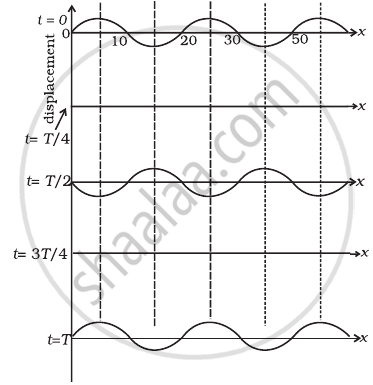Exercises | Q 15.29 | Page 111

The pattern of standing waves formed on a stretched string at two instants of time are shown in figure. The velocity of two waves superimposing to form stationary waves is 360 ms–1 and their frequencies are 256 Hz.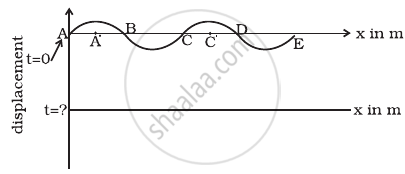1. Calculate the time at which the second curve is plotted.
2. Mark nodes and antinodes on the curve.
3. Calculate the distance between A′ and C′.
Exercises | Q 15.30 | Page 111

A tuning fork vibrating with a frequency of 512 Hz is kept close to the open end of a tube filled with water (Figure). The water level in the tube is gradually lowered. When the water level is 17 cm below the open end, maximum intensity of sound is heard. If the room temperature is 20°C, calculate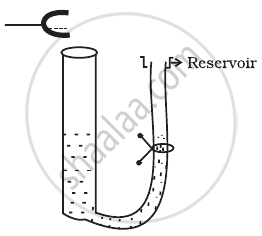1. speed of sound in air at room temperature
2. speed of sound in air at 0°C
3. if the water in the tube is replaced with mercury, will there be any difference in your observations?
Exercises | Q 15.31 | Page 111

Show that when a string fixed at its two ends vibrates in 1 loop, 2 loops, 3 loops and 4 loops, the frequencies are in the ratio 1:2:3:4.

#### LA

Exercises | Q 15.32 | Page 111

The earth has a radius of 6400 km. The inner core of 1000 km radius is solid. Outside it, there is a region from 1000 km to a radius of 3500 km which is in molten state. Then again from 3500 km to 6400 km the earth is solid. Only longitudinal (P) waves can travel inside a liquid. Assume that the P wave has a speed of 8 km s–1 in solid parts and of 5 km s–1 in liquid parts of the earth. An earthquake occurs at some place close to the surface of the earth. Calculate the time after which it will be recorded in a seismometer at a diametrically opposite point on the earth if wave travels along diameter?

Exercises | Q 15.33 | Page 112

If c is r.m.s. speed of molecules in a gas and v is the speed of sound waves in the gas, show that c/v is constant and independent of temperature for all diatomic gases.

Exercises | Q 15.34 | Page 112

Given below are some functions of x and t to represent the displacement of an elastic wave.

1. y = 5 cos (4x) sin (20t)
2. y = 4 sin (5x – t/2) + 3 cos (5x – t/2)
3. y = 10 cos [(252 – 250) πt] cos [(252 + 250)πt]
4. y = 100 cos (100πt + 0.5x)

State which of these represent

1. a travelling wave along –x direction
2. a stationary wave
3. beats
4. a travelling wave along +x direction.

Exercises | Q 15.35 (a) | Page 112

In the given progressive wave y = 5 sin (100 πt – 0.4 πx) where y and x are in m, t is in s. What is the amplitude.

Exercises | Q 15.35 (b) | Page 112

In the given progressive wave y = 5 sin (100 πt – 0.4 πx) where y and x are in m, t is in s. What is the wavelength.

Exercises | Q 15.35 (c) | Page 112

In the given progressive wave y = 5 sin (100 πt – 0.4 πx) where y and x are in m, t is in s. What is the frequency.

Exercises | Q 15.35 (d) | Page 112

In the given progressive wave y = 5 sin (100 πt – 0.4 πx) where y and x are in m, t is in s. What is the wave velocity.

Exercises | Q 15.35 (e) | Page 112

In the given progressive wave y = 5 sin (100 πt – 0.4 πx) where y and x are in m, t is in s. What is the particle velocity amplitude..

Exercises | Q 15.36 (a) | Page 112

For the harmonic travelling wave y = 2 cos 2π (10t – 0.0080x + 3.5) where x and y are in cm and t is second. What is the phase difference between the oscillatory motion at two points separated by a distance of 4 m.

Exercises | Q 15.36 (b) | Page 112

For the harmonic travelling wave y = 2 cos 2π (10t – 0.0080x + 3.5) where x and y are in cm and t is second. What is the phase difference between the oscillatory motion at two points separated by a distance of 0.5 m

Exercises | Q 15.36 (c) | Page 112

For the harmonic travelling wave y = 2 cos 2π (10t – 0.0080x + 3.5) where x and y are in cm and t is second. What is the phase difference between the oscillatory motion at two points separated by a distance of λ/2

Exercises | Q 15.36 (d) | Page 112

For the harmonic travelling wave y = 2 cos 2π (10t – 0.0080x + 3.5) where x and y are in cm and t is second. What is the phase difference between the oscillatory motion at two points separated by a distance of (3λ)/4 (at a given instant of time)

Exercises | Q 15.36 (e) | Page 112

For the harmonic travelling wave y = 2 cos 2π (10t – 0.0080x + 3.5) where x and y are in cm and t is second. What is the phase difference between the oscillatory motion at two points separated by a distance of What is the phase difference between the oscillation of a particle located at x = 100 cm, at t = T s and t = 5 s?

## Solutions for Chapter 15: Waves

Exercises## NCERT Exemplar solutions for Class 11 Physics chapter 15 - Waves

Shaalaa.com has the CBSE Mathematics Class 11 Physics CBSE solutions in a manner that help students grasp basic concepts better and faster. The detailed, step-by-step solutions will help you understand the concepts better and clarify any confusion. NCERT Exemplar solutions for Mathematics Class 11 Physics CBSE 15 (Waves) include all questions with answers and detailed explanations. This will clear students' doubts about questions and improve their application skills while preparing for board exams.

Further, we at Shaalaa.com provide such solutions so students can prepare for written exams. NCERT Exemplar textbook solutions can be a core help for self-study and provide excellent self-help guidance for students.

Concepts covered in Class 11 Physics chapter 15 Waves are Speed of Wave Motion, Reflection of Transverse and Longitudinal Waves, Displacement Relation for a Progressive Wave, The Speed of a Travelling Wave, Principle of Superposition of Waves, Introduction of Reflection of Waves, Standing Waves and Normal Modes, Beats, Doppler Effect, Wave Motion.

Using NCERT Exemplar Class 11 Physics solutions Waves exercise by students is an easy way to prepare for the exams, as they involve solutions arranged chapter-wise and also page-wise. The questions involved in NCERT Exemplar Solutions are essential questions that can be asked in the final exam. Maximum CBSE Class 11 Physics students prefer NCERT Exemplar Textbook Solutions to score more in exams.

Get the free view of Chapter 15, Waves Class 11 Physics additional questions for Mathematics Class 11 Physics CBSE, and you can use Shaalaa.com to keep it handy for your exam preparation.